#### You may also like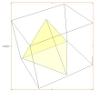### A Mean Tetrahedron

Can you number the vertices, edges and faces of a tetrahedron so that the number on each edge is the mean of the numbers on the adjacent vertices and the mean of the numbers on the adjacent faces?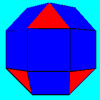### Rhombicubocts

Each of these solids is made up with 3 squares and a triangle around each vertex. Each has a total of 18 square faces and 8 faces that are equilateral triangles. How many faces, edges and vertices does each solid have?### Icosian Game

This problem is about investigating whether it is possible to start at one vertex of a platonic solid and visit every other vertex once only returning to the vertex you started at.

# Triangles to Tetrahedra

##### Age 11 to 14Challenge Level
What is a tetrahedron? Here are four different triangles made from Polydron (plastic shapes which click together):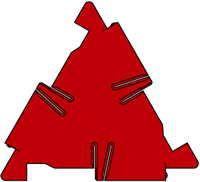Small equilateral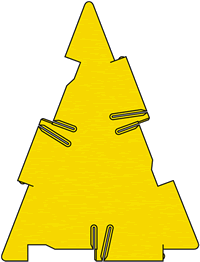Isosceles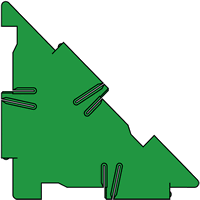Right angled isosceles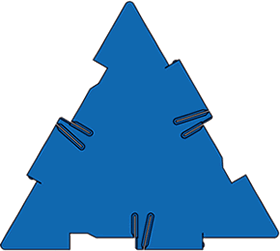Large equilateral

The sides of the small equilateral triangle are the same length as the short side of the isosceles triangle and the short sides of the right-angled isosceles triangle.

The sides of the large equilateral triangle are the same length as the long sides of the isosceles triangle and the long side of the right-angled isosceles triangle.

You have an unlimited number of each type of triangle.

How many different tetrahedra can you make? Convince us you have found them all.

Images reproduced with kind permission from Polydron.